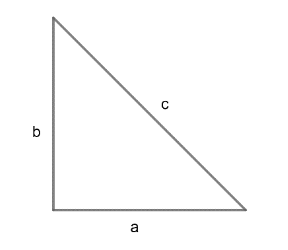Courses
Courses for Kids
Free study material
Free LIVE classes
MoreLIVE
Join Vedantu’s FREE Mastercalss

# In a right – angled triangle, the sided are $a,b\text{ and }c,$ with $c$ as hypotenuse and$c-b\ne 1,c+b\ne 1.$ Then the value of $\dfrac{\left( {{\log }_{c+b}}a+{{\log }_{c-b}}a \right)}{\left(2{{\log }_{c+b}}a\times {{\log }_{c-b}}a \right)}$A. $2$B. $-1$C. $\dfrac{1}{2}$D. $1$Verified
362.7k+ views
Hint: Use ${{\log }_{a}}b=\dfrac{1}{{{\log }_{b}}a}$ and basic formula of right angle
triangle.

As we have given that $a,b\text{ and }c$are sides of right – angled triangle with $c$ as
hypotenuse.By Pythagoras theorem:
${{c}^{2}}={{b}^{2}}+{{a}^{2}}..........\left( i \right)$
The given expression of which we need to find value is:
\begin{align} & =\dfrac{{{\log }_{c+b}}a+{{\log }_{c-b}}a}{2{{\log }_{c+b}}a\times {{\log }_{c-b}}a} \\ & =\dfrac{{{\log }_{c+b}}a}{2{{\log }_{c+b}}a\times {{\log }_{c-b}}a}+\dfrac{{{\log }_{c-b}}a}{2{{\log }_{c+b}}a\times {{\log }_{c-b}}a} \\ & =\dfrac{1}{2{{\log }_{c-b}}a}+\dfrac{1}{2{{\log }_{c+b}}a} \\ & =\dfrac{1}{2}\left( {{\log }_{a}}\left( c-b \right)+{{\log }_{a}}\left( c+b \right) \right)\text{ }\because \left( {{\log }_{a}}b=\dfrac{1}{{{\log }_{b}}a} \right) \\ & =\dfrac{1}{2}{{\log }_{a}}\left( c-b \right)\left( c+b \right)\text{ }\because \left( {{\log }_{x}}a+{{\log }_{x}}b={{\log }_{x}}ab \right) \\ & =\dfrac{1}{2}{{\log }_{a}}{{c}^{2}}-{{b}^{2}}.................\left( ii \right) \\ \end{align}
From the equation (i)
${{c}^{2}}={{b}^{2}}+{{a}^{2}}$
Hence, equation (ii) will become

\begin{align} & =\dfrac{1}{2}lo{{a}_{a}}{{a}^{2}} \\ & =\dfrac{2}{2}{{\log }_{a}}a\text{ }\left( {{\log }_{x}}{{a}^{n}}=n{{\log }_{x}}a \right) \\ & ={{\log }_{a}}a \\ & =1 \\ \end{align}
Hence, option D is the correct answer.
Note: (i) One need to remember the basic formula between logarithmic functions as used in
the solution for the flexibility which is an important part of the solution with respect to the
calculation point of view.
(ii) One can change the given expression in following way:
\begin{align} & \dfrac{{{\log }_{c+b}}a+{{\log }_{c-b}}a}{2{{\log }_{c+b}}a.{{\log }_{c-b}}a} \\ & =\dfrac{\dfrac{{{\log }_{x}}a}{{{\log }_{x}}\left( c+b \right)}+\dfrac{{{\log }_{x}}a}{{{\log }_{x}}\left( c-b \right)}}{\dfrac{2{{\log }_{x}}a}{{{\log }_{x}}\left( c+b \right)}\times \dfrac{{{\log }_{x}}a}{{{\log }_{x}}\left( c-b \right)}} \\ \end{align}
As we have formula ${{\log }_{a}}b=\dfrac{{{\log }_{c}}b}{{{\log }_{c}}a}$ with positive $c$.
And simplifying the above expression will also give the answer, but at the end the same
calculation is required. So no need to change the bases of given logarithm functions. We can
observe the expression and need to solve in the same way as done in the solution.
Last updated date: 22nd Sep 2023
Total views: 362.7k
Views today: 10.62k